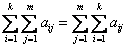## I get confused with double summation!

Double sum is nothing more than sum of a sum. If the elements of the sum have two indices and you want to add the index one by one, then you use double sums. In calculating double summations, here are the steps

1. First, the outer-sum index is hold and increment the inner index
2. After all the inner-sum index has been used, then increment the outer-sum index.
3. Repeat the two steps above for the entire outer-sum index.

It is easier to see the steps above by example below. Before that, you may remember one nice property of double summation:

We can interchange order of double summationExample

We have matrix< Previous | Next | Contents >

See Also: Kardi Teknomo's Tutorial

Preferable reference for this tutorial is

Teknomo, Kardi (2015) Summation Tutorial. http://people.revoledu.com/kardi/tutorial/BasicMath/Sum/